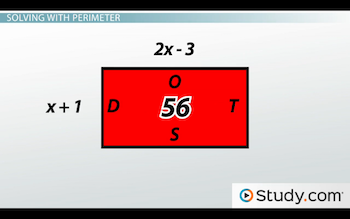# Perimeter of Triangles and Rectangles

An error occurred trying to load this video.

Try refreshing the page, or contact customer support.

Coming up next: Area of Triangles and Rectangles

### You're on a roll. Keep up the good work!

Replay
Your next lesson will play in 10 seconds
• 0:05 The Perimeter
• 0:47 Triangle & Rectangle…
• 3:47 Solving with Perimeter
• 8:02 Lesson Summary
Save Save

Want to watch this again later?

Timeline
Autoplay
Autoplay
Speed Speed Audio mode

#### Recommended Lessons and Courses for You

Lesson Transcript
Instructor: DaQuita Hester

DaQuita has taught high school mathematics for six years and has a master's degree in secondary mathematics education.

Without realizing it, we calculate and use the perimeter of triangles and rectangles in regular everyday situations. Learn more about the perimeter of triangles and rectangles in this lesson, and test your knowledge with a quiz.

## The Perimeter

As part of a new fitness program, Melody has to walk around her block three times every day. If each side of her block is 80 ft long, how many feet will she walk every day?

To answer this question, we must find out how many feet are around her entire block, not just one side. This concept is known as the perimeter, which is defined as the distance around an object, shape or figure. To find the perimeter of any polygon, all we have to do is add up the length of all the sides. For this lesson, we will focus on the perimeter of triangles and rectangles, and we'll look at a few examples, beginning with Melody.

## Triangle and Rectangle Perimeters

Recall that Melody has to walk around her block three times every day, and each side of her block is 80 ft long. To determine the total length of her walk, we must figure out how many feet are around the entire block. To do so, we'll add 80 + 80 + 80 + 80, since there are four sides to her block. Alternatively, since all of the sides of her block are the same length, we could also multiply 4 * 80. Using either method, we will see that the perimeter of Melody's block is 320. Since she has to circle the block three times, we will add 320 + 320 + 320 to get a total of 960. So, in conclusion, Melody will walk 960 ft every day for her fitness program.

Let's look at another.

Daniel has 25 ft of decorative border for his rectangular bulletin board. If the width of his board is 3 ft and the length of his board is 10 ft, does he have enough border to line the entire board?

To determine if Daniel has enough border, we must calculate the perimeter of his board. Since it's a rectangle, we know that both widths and both lengths will be the same. Therefore, to find the perimeter, we can either add 3 + 3 + 10 + 10 or we can multiply 2 * 3 and 2 * 10 and then add 6 + 20. No matter which method we use, the perimeter of the board is 26 ft. Since Daniel only has 25 ft of border, he does not have enough to line the entire board.

Here's one last example.

Christy is creating a triangular collage of pictures. One side of the collage is 12 in and the other two sides are 18 in. If she wants to frame the collage with ribbon, how much ribbon will she need? And, if the ribbon costs \$0.75 per foot, how much will the ribbon cost her?

To determine the amount of ribbon that Christy needs, we must first figure out the perimeter of her collage. To do so, let's add up the length of all the sides of the triangle: 12 + 18 + 18. Simplifying this calculation leaves us with a perimeter of 48. Therefore, Christy needs 48 in. of ribbon to frame her collage.

Now, let's determine the cost. Recalling that 12 in = 1 ft, we can conclude that 48 in = 4 ft. Since the ribbon costs \$0.75 per foot, let's multiply 4 * \$0.75. Doing so gives a total of \$3. In conclusion, Christy will spend \$3 on ribbon for her collage.

## Solving with Perimeter

In all of these examples, we were given the measure of each side to calculate the perimeter. Now, let's look at how we can use perimeter to find the measure of the sides.

Here's rectangle DOTS, which has a perimeter of 56:If the length of the rectangle is 2x - 3, and the width of the rectangle is x + 1, how long is the rectangle?

To unlock this lesson you must be a Study.com Member.

### Register to view this lesson

Are you a student or a teacher?

#### See for yourself why 30 million people use Study.com

##### Become a Study.com member and start learning now.
Back
What teachers are saying about Study.com

### Earning College Credit

Did you know… We have over 200 college courses that prepare you to earn credit by exam that is accepted by over 1,500 colleges and universities. You can test out of the first two years of college and save thousands off your degree. Anyone can earn credit-by-exam regardless of age or education level.